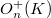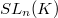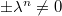﻿

### The Maximality of the Group of Euclidean Similarities within the Affine Group II

#### Abstract

The purpose of this note is to show that for an arbitrary Pythagorean field$K$ the group$O^+_n(K)$ is maximal within$SL_n(K)$ if, and only if,$K$ admits only of Archimedean orderings.Under the same conditions the group of$n-$dimensional Euclidean similarities is maximal within the group of all affine mappings having a determinant of the form$\pm\lambda^n \not=0$.

DOI Code: 10.1285/i15900932v21n2p135

Full Text: PDF